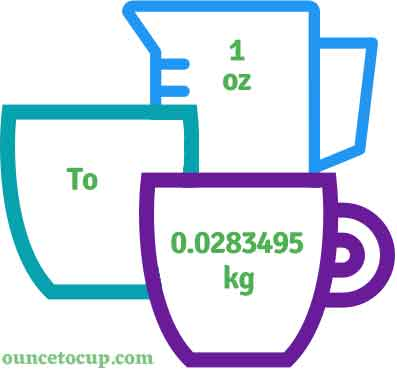# Ounce to Kilogram (oz to kg conversion)

Are you cooking your favorite dish? The detailed chart in the recipe includes the calculation of 1 ounces to kg conversion? Do not worry; check this conversion tool to find how many 1 ounces equal to kg in a minute. This 1 oz to kg converter gives an exact measurement for any recipe you prepare.

Ounce Value:

oz

Kilogram Value:

kg

1 Ounce = 0.0283495 Kilogram
(1 oz = 0.0283495 kg)

Try our auto 1 ounce to kilogram calculator (Without Convert Button), Just change the first field value and you got final value.## How many kg is a oz?

We know that the mass value of 1 oz is equal to 0.0283495 kg. If you want to convert 1 fluid oz to an equal number of kg, just divide the mass value by 35.274. Hence, 1 Ounce is equal to 0.0283495 kg.

The Answer is: 1 US Fluid Ounces = 0.0283495 US kg

1 oz = 0.0283495 kg

Many of them try to search or find an answer for what is 1 ounces in kg? So, we’ll start with 1 fl to oz kg conversion to know how big is 1 oz.

## How To Calculate 1 fluid oz to kg?

To calculate 1 fluid ounces to an equal number of kilogram, simply follow the steps below.

Fluid Ounces to Kilograms formula is:

Kilogram = Fluid Ounce / 35.274

Assume that we are finding out how many kg were found in 1 fl oz of water, divide by 35.274 to get the result.

Applying to Formula: kg = 1 oz / 35.274 = 0.0283495 kg.

## How To Convert oz to kg?

• To convert fluid ounces to kg,
• Simply divide the fluid ounce value by 35.274.
• Applying to the formula, kg = 1 ounces / 35.274 [1/35.274].
• Hence, 1 ounces is equal to 0.0283495 kg.

## Some quick table references for ounce to kilogram conversions:

Ounce [oz]Kilogram [kg]
1 oz0.0283 kg
2 oz0.0567 kg
3 oz0.085 kg
4 oz0.1134 kg
5 oz0.1417 kg
6 oz0.1701 kg
7 oz0.1984 kg
8 oz0.2268 kg
9 oz0.2551 kg
10 oz0.2835 kg
11 oz0.3118 kg
12 oz0.3402 kg
13 oz0.3685 kg
14 oz0.3969 kg
15 oz0.4252 kg

## Reverse Calculation: How many ounces are in a kg?

• To convert 1 kg to oz,
• Simply multiply the 1 kg by 35.274.
• Then, applying the formula, ounce = 1 kg * 35.274 [1x35.274 = 35.274].
• Hence, 1 kg is equal to 35.274 oz.

### Related Converter:

Formula: Ounce to Kilogram

kg = ounce / 35.274

Applying to Formula,

kg = 1/35.274 = 0.0283495

1 oz = 0.0283495 kg